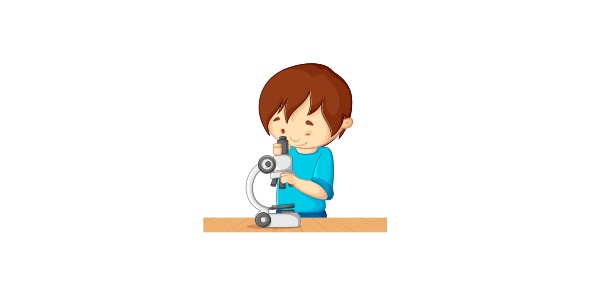# Have Difficulty In 11th Grade Economics? Here Is A Practice Test!

26 Questions | Total Attempts: 1086SettingsEconomics is the social science that studies how people interact with value; in particular, the production, distribution, and consumption of goods and services. Have Difficulty in 11th Grade Economics? Here Is a Practice Test for you

• 1.
Material desires of individuals or communitys can be defined as
• A.

Needs

• B.

Demands

• C.

Wants

• D.

Opportunity costs

• 2.
Desires of each person are
• A.

Collective wants

• B.

Individual wants

• C.

Demands

• 3.
A library is an example of :
• A.

Individual want

• B.

Collective want

• C.

Demand

• 4.
Pick the recurrent want (pick all that apply)
• A.

A new car

• B.

Clothes

• C.

Food

• D.

Petrol

• 5.
What factors affect wants (tick all that apply)
• A.

Income

• B.

Age

• C.

Fashion

• D.

Technology

• 6.
Which of these is not an example of opportunity costs
• A.

Paying \$300 for a mobile phone

• B.

Missing a basket ball game to go to the movies

• C.

Miss a basket ball game because the train was late

• 7.
What are the four key economic issues
• A.

How to produce

• B.

What to produce

• C.

How much to produce

• D.

How to distribute

• E.

Why to produce

• 8.
Items that have not been produced for immediate consumption but will be used for the production of other goods are termed as
• A.

Consumer goods

• B.

Capital goods

• C.

Services

• 9.
Which one of these is a capital good? (tick all that apply)
• A.

Labour

• B.

Machinery

• C.

Truck

• 10.
Demand by all consumers for a particular good or service.
• A.

• 11.
What is NOT a factor influencing market demand
• A.

Price of good or service itself

• B.

Changes in consumer taste and preferences

• C.

Past prices

• D.

Level of income

• 12.
The way the economy income is spread among the members of different social and socio economic groups is termed?
• A.

Income distribution

• B.

Social income

• C.

Economic income

• D.

Income allocation

• 13.
What does "Ceteris paribus assumption" mean
• A.

Assumption used in economics to isolate relationship between two economic variables

• B.

Assumption used in economics to describe income patterns

• C.

Assumption used in economics to compare the relationship between income and spending

• 14.
The law of demand – states
• A.

That the quantity demanded by consumers falls as the price rises

• B.

That the quantity demanded by consumers rises as the price rises

• C.

That the quantity demanded by consumers fall as the price falls

• 15.
When a decrease in the price of good or service causes an increase in the quantity demanded this is termed as
• A.

• 16.
What are other factors that affect demand beside price (tick all that apply)
• A.

Expected future prices

• B.

Changes in consumer taste and preferences

• C.

Price of substitute goods

• D.

None of these

• 17.
Elastic demand is which of the following?
• A.

Weak response to a price change

• B.

Strong response to a price change

• C.

No response to a price change

• 18.
Contraction of demand is
• A.

Strong response to a price change

• B.

Weak response to a price change

• C.

When an increase in the price of good or service causes an decrease in the quantity demanded.

• 19.
A weak response to a price change is termed as
• A.

Elastic demand

• B.

Inelastic demand

• C.

Unit elastic demand

• 20.
The law of demand states
• A.

That as the price of a good decreases, the quantity demanded will decrease

• B.

That as the price of a good increases, the quantity demanded will increase

• C.

That as the price of a good increases, the quantity demanded will decrease

• 21.
The total stock of goods and services held by a firm at a particular time, which is intended for sale to consumers is termed
• A.

Supply

• B.

Inventory

• C.

Goods

• D.

Demand

• 22.
Price mechanism determines what?
• A.

The equilibrium in supply

• B.

The equilibrium in demand

• C.

The equilibrium in the market

• 23.
The non cash exchange of goods and services is known as
• A.

Exchanging

• B.

Bartering

• C.

Swapping

• 24.
What is recession
• A.

Stage of the business cycle where there is no economic activity

• B.

Stage of the business cycle where there is increasing economic activity

• C.

Stage of the business cycle where there is decreasing economic activity

• 25.
Human effort, both physical and mental used to produce goods and services is termed as
• A.

Capital

• B.

Labour

• C.

Natural resources

Related TopicsBack to top